March 4, 2020

## Presentations

• 4.17 & 4.19 Banu Boopalan
• 4.1 & 4.21 Subhalaxmi Rout
• 4.45 Vinayak Kamath
• 4.35 Jeff Shamp

## Population Distribution (Uniform)

n <- 1e5
pop <- runif(n, 0, 1)
mean(pop)
##  0.5008915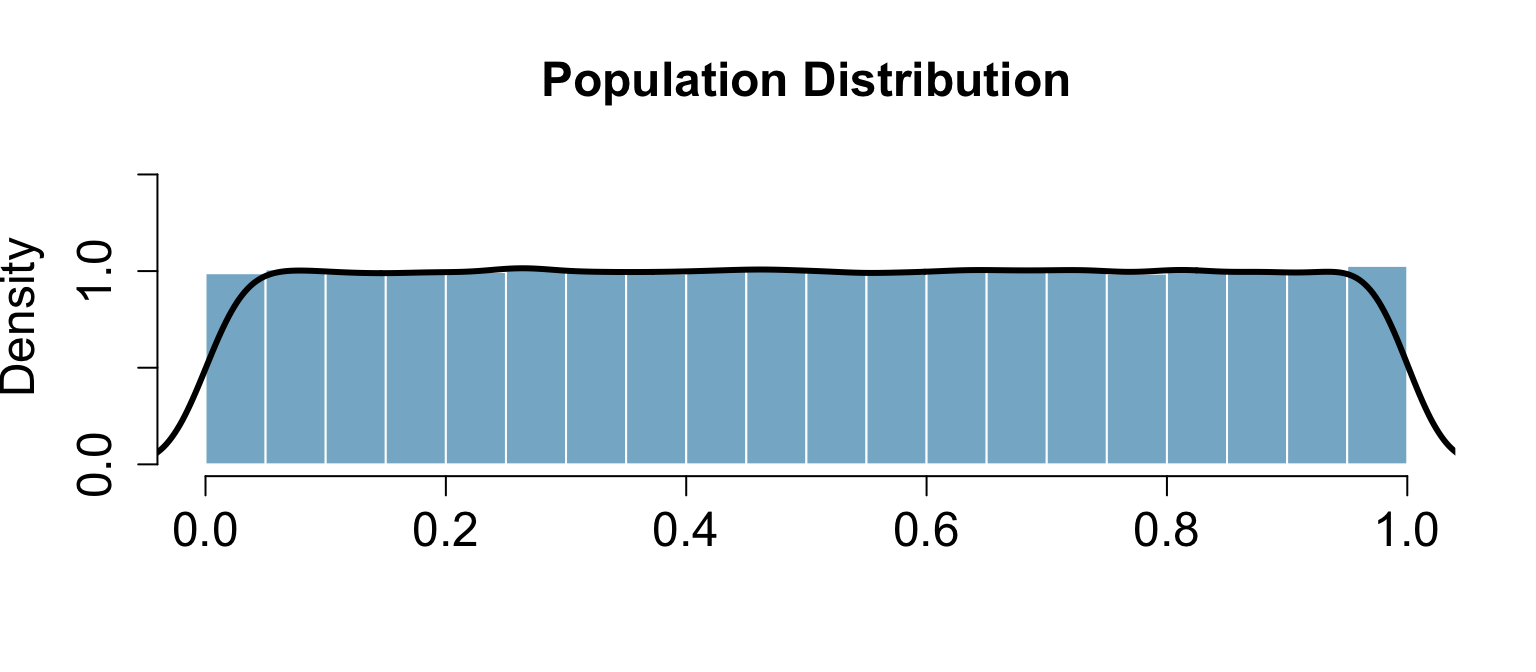## Random Sample (n=10)

samp1 <- sample(pop, size=10)
mean(samp1)
##  0.5462923
hist(samp1)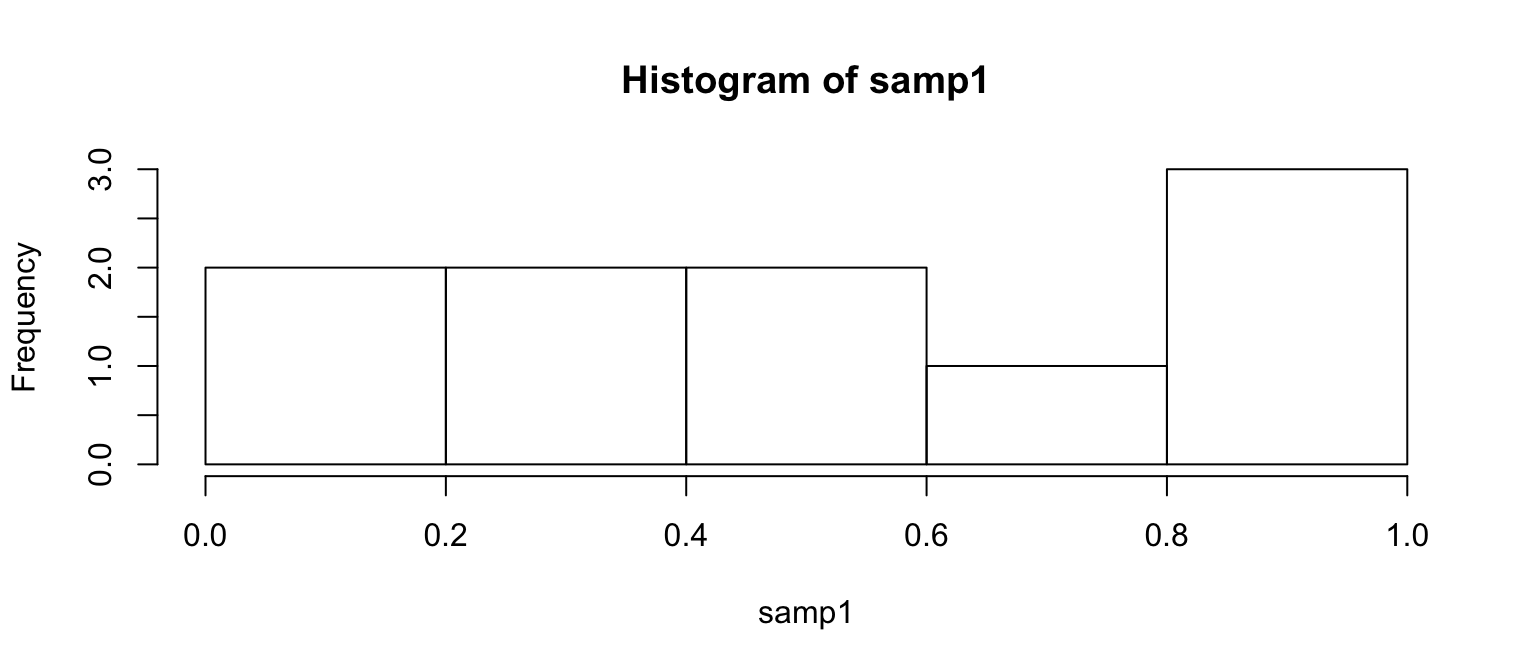## Random Sample (n=30)

samp2 <- sample(pop, size=30)
mean(samp2)
##  0.5130467
hist(samp2)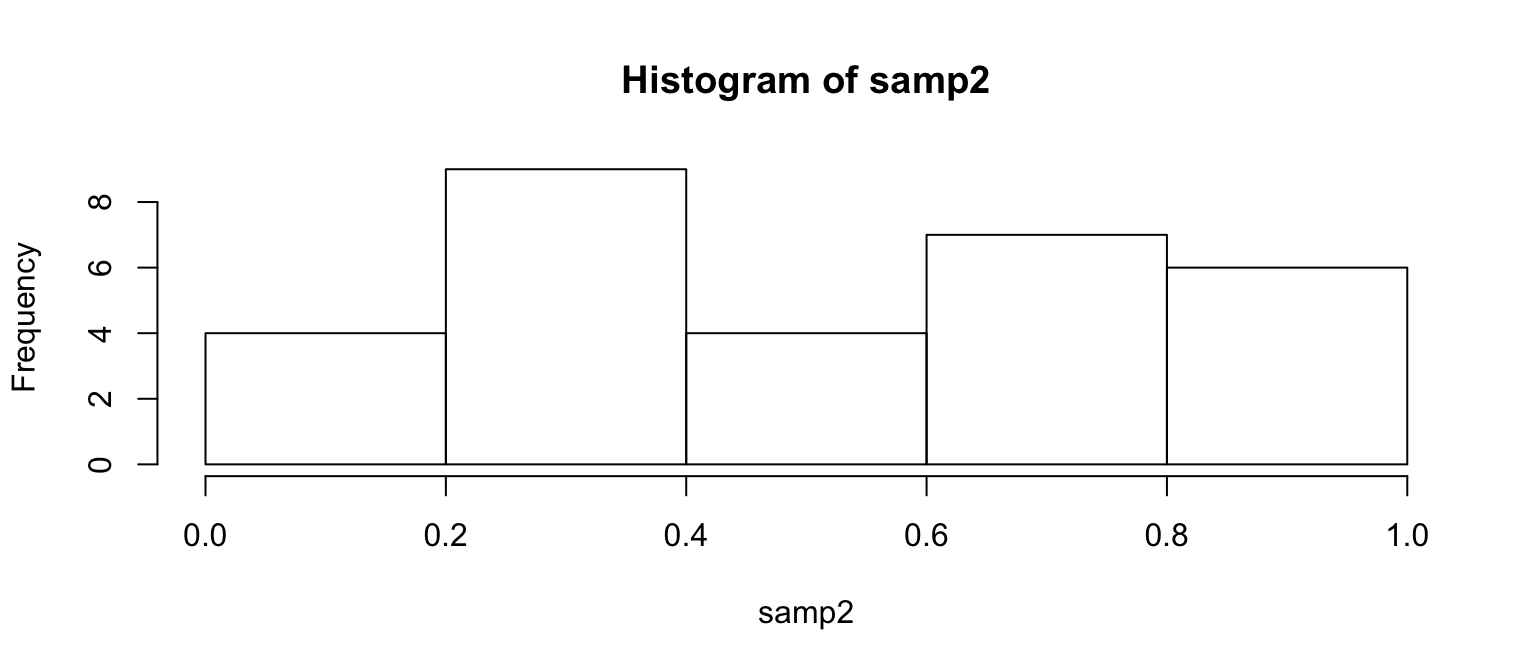## Lots of Random Samples

M <- 1000
samples <- numeric(length=M)
for(i in seq_len(M)) {
samples[i] <- mean(sample(pop, size=30))
}
head(samples, n=8)
##  0.5314329 0.4892158 0.5300205 0.5349329 0.5290058 0.4482886 0.3628467
##  0.4448132

## Sampling Distribution

hist(samples)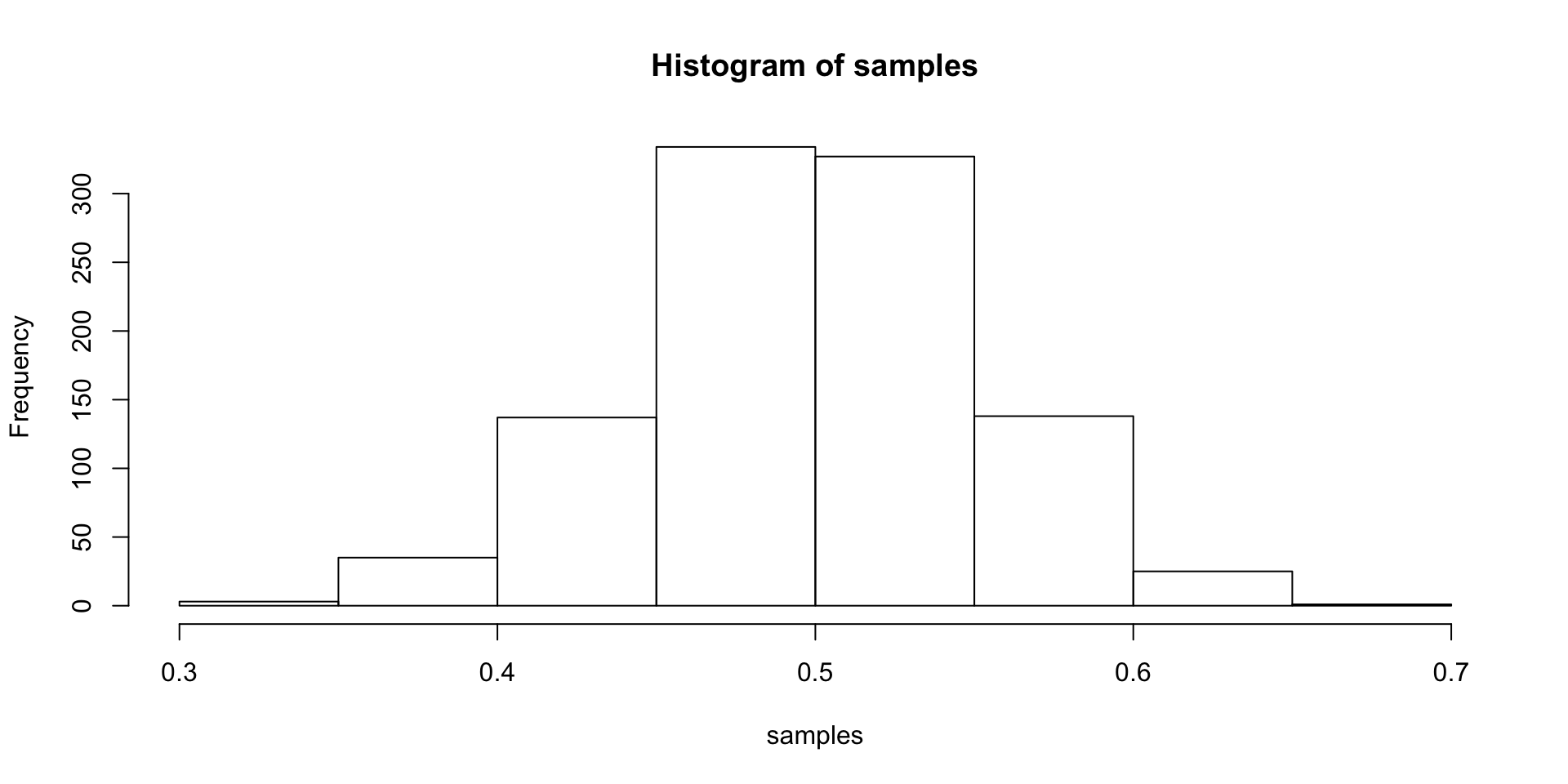## Central Limit Theorem (CLT)

Let $$X_1$$, $$X_2$$, …, $$X_n$$ be independent, identically distributed random variables with mean $$\mu$$ and variance $$\sigma^2$$, both finite. Then for any constant $$z$$,

$\underset { n\rightarrow \infty }{ lim } P\left( \frac { \bar { X } -\mu }{ \sigma /\sqrt { n } } \le z \right) =\Phi \left( z \right)$

where $$\Phi$$ is the cumulative distribution function (cdf) of the standard normal distribution.

## In other words…

The distribution of the sample mean is well approximated by a normal model:

$\bar { x } \sim N\left( mean=\mu ,SE=\frac { \sigma }{ \sqrt { n } } \right)$

where SE represents the standard error, which is defined as the standard deviation of the sampling distribution. In most cases $$\sigma$$ is not known, so use $$s$$.

## CLT Shiny App

shiny_demo('sampdist')
shiny_demo('CLT_mean')

## Standard Error

samp2 <- sample(pop, size=30)
mean(samp2)
##  0.5410922
(samp2.se <- sd(samp2) / sqrt(length(samp2)))
##  0.05035122

## Confidence Interval

The confidence interval is then $$\mu \pm CV \times SE$$ where CV is the critical value. For a 95% confidence interval, the critical value is ~1.96 since

$\int _{ -1.96 }^{ 1.96 }{ \frac { 1 }{ \sigma \sqrt { 2\pi } } { d }^{ -\frac { { \left( x-\mu \right) }^{ 2 } }{ 2{ \sigma }^{ 2 } } } } \approx 0.95$

qnorm(0.025) # Remember we need to consider the two tails, 2.5% to the left, 2.5% to the right.
##  -1.959964
(samp2.ci <- c(mean(samp2) - 1.96 * samp2.se, mean(samp2) + 1.96 * samp2.se))
##  0.4424038 0.6397806

## Confidence Intervals (cont.)

We are 95% confident that the true population mean is between 0.4424038, 0.6397806.

That is, if we were to take 100 random samples, we would expect at least 95% of those samples to have a mean within 0.4424038, 0.6397806.

ci <- data.frame(mean=numeric(), min=numeric(), max=numeric())
for(i in seq_len(100)) {
samp <- sample(pop, size=30)
se <- sd(samp) / sqrt(length(samp))
ci[i,] <- c(mean(samp),
mean(samp) - 2 * se,
mean(samp) + 2 * se)
}
ci$sample <- 1:nrow(ci) ci$sig <- ci$min < 0.5 & ci$max > 0.5

## Confidence Intervals

ggplot(ci, aes(x=min, xend=max, y=sample, yend=sample, color=sig)) +
geom_vline(xintercept=0.5) +
geom_segment() + xlab('CI') + ylab('') +
scale_color_manual(values=c('TRUE'='grey', 'FALSE'='red'))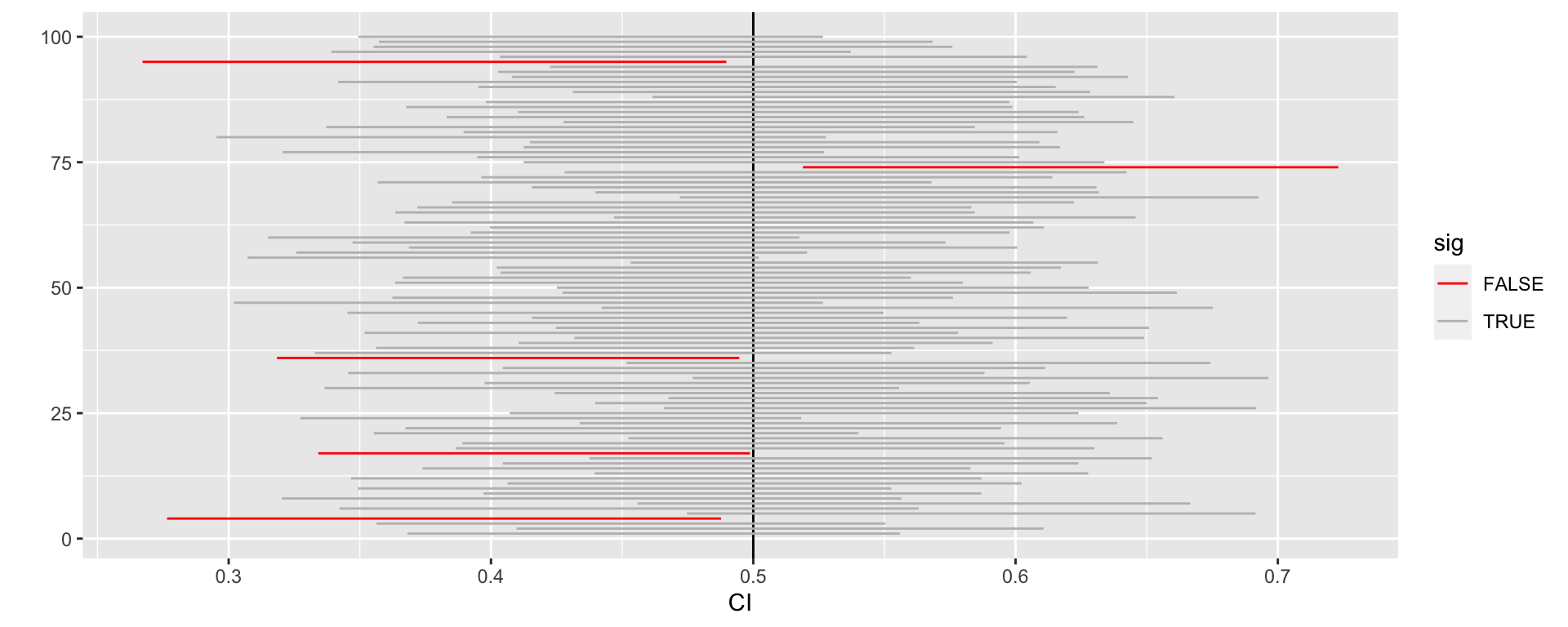## Hypothesis Testing

• We start with a null hypothesis ($$H_0$$) that represents the status quo.
• We also have an alternative hypothesis ($$H_A$$) that represents our research question, i.e. what we???re testing for.
• We conduct a hypothesis test under the assumption that the null hypothesis is true, either via simulation or traditional methods based on the central limit theorem.
• If the test results suggest that the data do not provide convincing evidence for the alternative hypothesis, we stick with the null hypothesis. If they do, then we reject the null hypothesis in favor of the alternative.

## Hypothesis Testing (using CI)

$$H_0$$: The mean of samp2 = 0.5
$$H_A$$: The mean of samp2 $$\ne$$ 0.5

Using confidence intervals, if the null value is within the confidence interval, then we fail to reject the null hypothesis.

(samp2.ci <- c(mean(samp2) - 2 * sd(samp2) / sqrt(length(samp2)),
mean(samp2) + 2 * sd(samp2) / sqrt(length(samp2))))
##  0.4403897 0.6417946

Since 0.5 fall within 0.4403897, 0.6417946, we fail to reject the null hypothesis.

## Hypothesis Testing (using p-values)

$\bar { x } \sim N\left( mean=0.49,SE=\frac { 0.27 }{ \sqrt { 30 } = 0.049 } \right)$

$Z=\frac { \bar { x } -null }{ SE } =\frac { 0.49-0.50 }{ 0.049 } = -.204081633$

pnorm(-.204) * 2
##  0.8383535

## Hypothesis Testing (using p-values)

normalPlot(bounds=c(-.204, .204), tails=TRUE)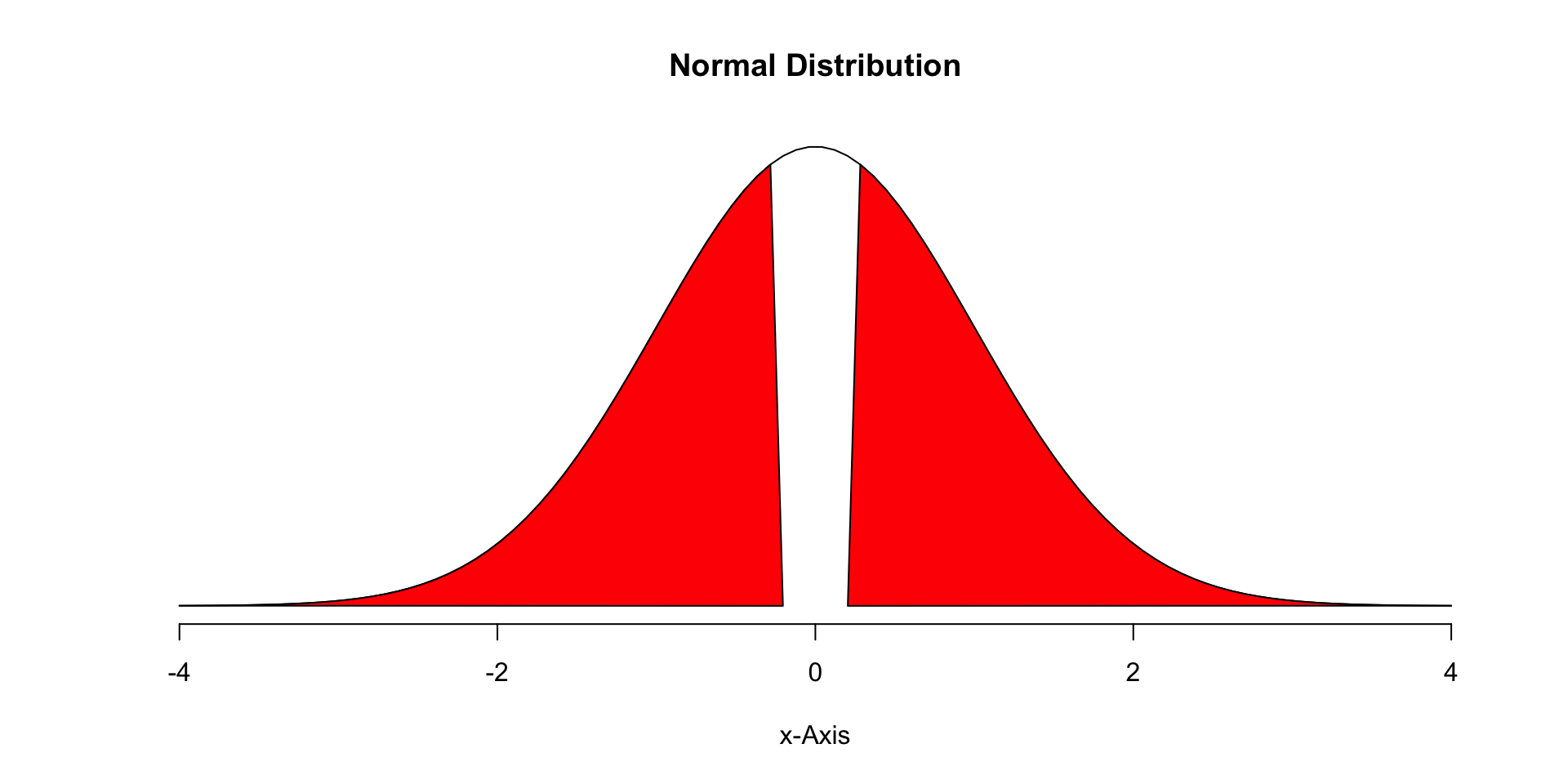## Type I and II Errors

There are two competing hypotheses: the null and the alternative. In a hypothesis test, we make a decision about which might be true, but our choice might be incorrect.

fail to reject H0 reject H0
H0 true Type I Error
HA true Type II Error

• Type I Error: Rejecting the null hypothesis when it is true.
• Type II Error: Failing to reject the null hypothesis when it is false.

## Hypothesis Test

If we again think of a hypothesis test as a criminal trial then it makes sense to frame the verdict in terms of the null and alternative hypotheses:

H0 : Defendant is innocent
HA : Defendant is guilty

Which type of error is being committed in the following circumstances?

• Declaring the defendant innocent when they are actually guilty
Type 2 error
• Declaring the defendant guilty when they are actually innocent
Type 1 error

Which error do you think is the worse error to make?

## Null Distribution

(cv <- qnorm(0.05, mean=0, sd=1, lower.tail=FALSE))
##  1.644854
PlotDist(alpha=0.05, distribution='normal', alternative='greater')
abline(v=cv, col='blue')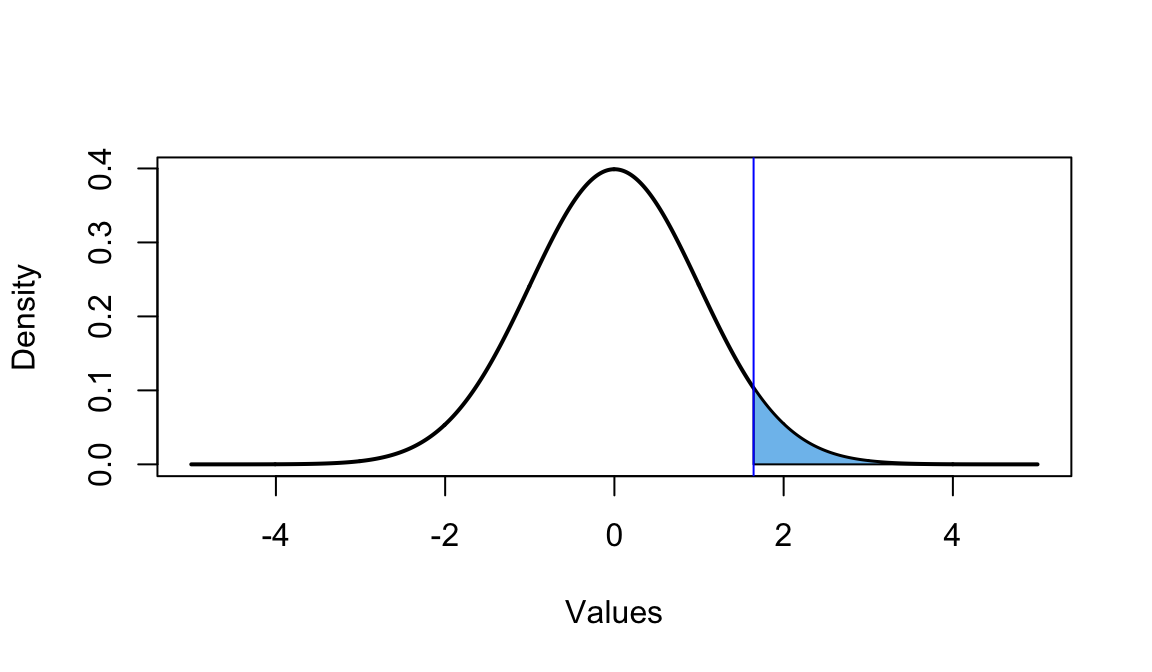## Alternative Distribution

cord.x1 <- c(-5, seq(from = -5, to = cv, length.out = 100), cv)
cord.y1 <- c(0, dnorm(mean=cv, x=seq(from=-5, to=cv, length.out = 100)), 0)
curve(dnorm(x, mean=cv), from = -5, to = 5, n = 1000, col = "black",
lty = 1, lwd = 2, ylab = "Density", xlab = "Values")
polygon(x = cord.x1, y = cord.y1, col = 'lightgreen')
abline(v=cv, col='blue')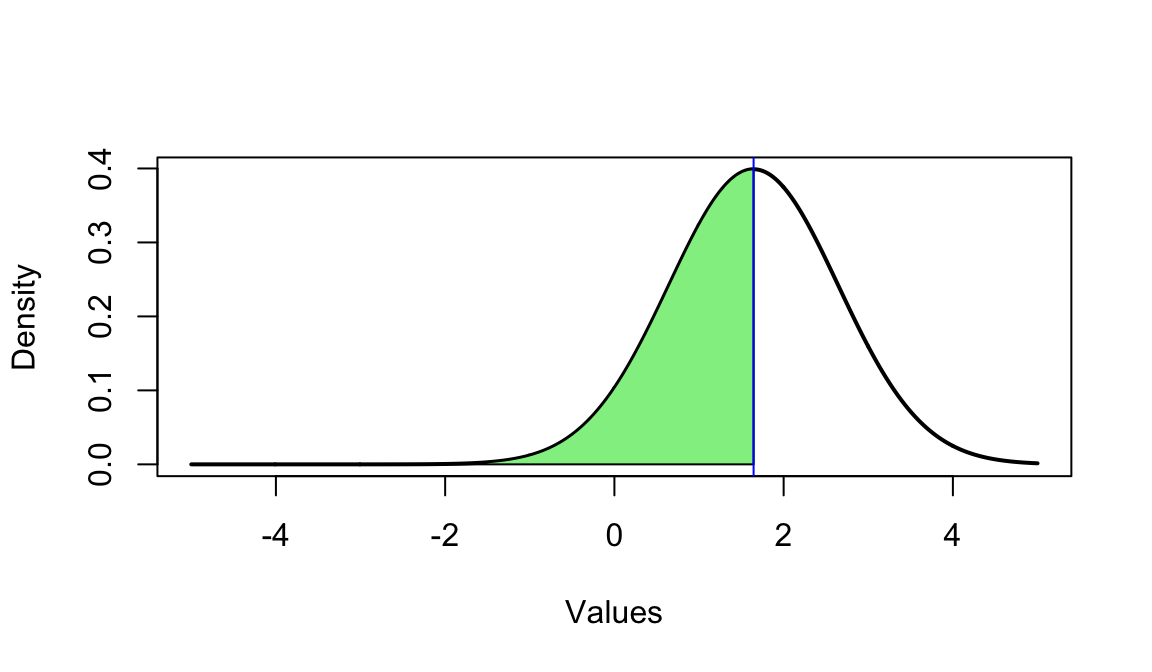pnorm(cv, mean=cv, lower.tail = FALSE)
##  0.5

## Another Example (mu = 2.5)

mu <- 2.5
(cv <- qnorm(0.05, mean=0, sd=1, lower.tail=FALSE))
##  1.644854